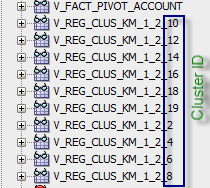## Friday, November 11, 2016

### Dynamic Data Sources Based on Cluster Rules

In the last post we saw how to run regression with Data Miner, interpret its results and how to use cluster information to filter regression data sources. It was quite a laborious task - even with a small number of clusters. If we want to experiment with the number of clusters, clustering methods and their parameters, and feed those clusters dynamically into regressions, we would need to run regressions programmatically.

Let’s start with a case of a simple regression ran by DBMS_DATA_MINING package. Again, I’ll refer the reader to “Predictive Analytics Using Oracle Data Miner” by Brendan Tierney or to his blog for step by step instructions of orchestrating data mining workflows with PL/SQL. Basically in order to run regression with PL/SQL instead of using DataMiner UI, you need to:

• Create a table for regression settings
• Populate that table with settings
• Define a data source (a view in our case - V_REGRESSION_ACC_002)
• Create the model with DBMS_DATA_MINING package
• Query results

 --Here’s the settings table CREATE TABLE REG_SETTING_002 (SETTING_NAME VARCHAR2(300), SETTING_VALUE VARCHAR2(4000)); Begin Insert into REG_SETTING_002 (SETTING_NAME,SETTING_VALUE) values (dbms_data_mining.algo_name,dbms_data_mining.algo_generalized_linear_model); commit; --Create the model SYS.DBMS_DATA_MINING.CREATE_MODEL( model_name=>'REGRESSION_ACC_002', mining_function=>dbms_data_mining.regression, data_table_name=>'V_REGRESSION_ACC_002', case_id_column_name=>'CASE_ID', target_column_name=>'12346_ACC', settings_table_name=>'REG_SETTING_002' ); end; /

The data source 'V_REGRESSION_ACC_002' would have a WHERE clause with cluster definition. In other words the following filter should be applied:

 And "BUSINESSUNIT" IN ('B_xxxx', 'B_xxxy', 'B_xxxz', ...) and "COSTCENTER" IN ('C_xxxx', 'C_xxxy', 'C_xxxz', ...)

How that cluster rule can be queried? I’ll refer to Brendan’s blog for rule query and to Tom Kyte blog for converting multiple rows into comma separated list. In our case the query for cluster 8 and rule for COSTCENTER dimension would be:

 with t1 as ( select t.id,         a.attribute_name,         a.conditional_operator,       nvl(a.attribute_str_value,       a.attribute_num_value) AS atrvalue,       a.attribute_support,       a.attribute_confidence from TABLE(dbms_data_mining.get_model_details_km('CLUS_KM_1_2'))  t,       TABLE(t.rule.antecedent)  a where 1=1 and t.id = 8 and a.attribute_name='COSTCENTER' ) SELECT SUBSTR (SYS_CONNECT_BY_PATH (atrvalue , ','), 2) csv      FROM (SELECT '"'||atrvalue||'"' atrvalue, ROW_NUMBER () OVER (ORDER BY atrvalue ) rn,                   COUNT (*) OVER () cnt              FROM t1)     WHERE rn = cnt START WITH rn = 1 CONNECT BY rn = PRIOR rn + 1;

This will give us something like this list (the actual cost center codes are obfuscated):

"C_7420","C_4348","C_8253","C_7356","C_1478","C_4445","C_2653","C_8621","C_7429","C_5718","C_7111","C_7212","C_7102","C_8214","C_7105","C_2582","C_8279","C_5403","C_3336","C_5494","C_1699","C_7808","C_2921","C_1443","C_4880","C_9425","C_1508","C_4529","C_9989","C_4884","C_6871","C_9206","C_2550","C_5658","C_4455","C_9869","C_5594","C_9288","C_6026","C_3045","C_9264","C_3173","C_3180","C_7550","C_4140","C_6560","C_6802","C_3717","C_4475","C_9420","C_4808","C_8949","C_9966","C_4891","C_5648","C_8109","C_1219","C_5860","C_5416","C_5696","C_1201","C_7483","C_5781","C_3341","C_8140","C_1848","C_1449","C_9547","C_3318","C_3972","C_8261","C_4860","C_6658","C_9257","C_2750","C_6091","C_6316","C_1552","C_1497","C_4278","C_9596","C_2607","C_6416","C_4446","C_8388","C_3870","C_8727","C_2083","C_5332","C_7126","C_6998","C_9682","C_5234","C_7094","C_5246","C_6978","C_4928","C_5578","C_8033","C_6197","C_6687","C_4818","C_3574","C_7374","C_4368","C_5672","C_7632","C_7728","C_4644","C_9988","C_2692","C_6409","C_8017","C_9386","C_9059","C_5214","C_9930","C_2506","C_7060","C_4264","C_5640","C_5915","C_1436","C_3991","C_2381","C_1571","C_2850","C_6293","C_3125","C_5657","C_2275","C_3646","C_7077","C_4886","C_1253","C_3164","C_3677","C_6162","C_8123","C_2249","C_9296","C_8628","C_5577","C_8541","C_4581","C_8933","C_1420","C_9861","C_1403","C_3078","C_1654","C_7759","C_7014","C_7262","C_5283","C_4714","C_9501","C_2733","C_9351","C_6007","C_8832"

Now we want to iterate through multiple clusters, create data sources and run regressions. For that purpose we could write a stored procedure that will use a cursor of level 0 clusters. To get a set of level 0 clusters we can use the following query (we’ll pass a model name as a parameter into procedure):

 SELECT ID FROM ( SELECT PARENT, ID, CONNECT_BY_ISLEAF ISLEAF, LEVEL CURRLEV FROM table(dbms_data_mining.get_model_details_km('CLUS_KM_1_2'))    CONNECT BY PRIOR ID= PARENT    START WITH NVL(PARENT,0) = 0    ) WHERE ISLEAF=1     ;

And the query to get a list of distinct attributes is:

 select distinct         a.attribute_name from TABLE(dbms_data_mining.get_model_details_km('CLUS_KM_1_2'))  t,       TABLE(t.rule.antecedent)  a where 1=1 and t.id = 8 and a.attribute_confidence>0 ;

Now by iterating through all the attributes and leaf clusters of a particular model we can create data sources for regression dynamically. We’ll use the following template to create the view:

 CREATE OR REPLACE VIEW V_REG_MODELTOKEN_CLUSTERTOKEN AS SQLTOKEN WHERE 1=1 CLUSTERFILTERTOKEN

MODELTOKEN,CLUSTERTOKEN,SQLTOKEN,CLUSTERFILTERTOKEN will be replaced in the stored procedure, and MODELTOKEN,SQLTOKEN will be passed into procedure as parameters.We will also need to define which dimension is a measure dimension used for dependent/independent variables in regression. In our case that is an account dimension. Since we pivoted that dimension (see previous post) and have accounts in columns, we can’t have a cluster rule (a where clause in other words) for measures dimension, so it is excluded from where clause.  In the end, when you run the following procedure, data sources for all clusters of a particular model should be created:

 DECLARE  VVIEWBASESQL CLOB;  CURR_MODEL VARCHAR2(200);  MEASURE_ATTR VARCHAR2(200); BEGIN  VVIEWBASESQL :=                'SELECT                "BUSINESSUNIT"||                "COSTCENTER"||                "CURRENCY"||                "ENTITY"||                "MNUM"||                "PCC"||                "YEAR" CASE_ID,                BUSINESSUNIT,                ENTITY,                PCC,                COSTCENTER,                SCENARIO,                VERSION,                YEAR,                HSP_RATES,                CURRENCY,                MNUM,                "12345_ACC",                "12346_ACC"                FROM T_FACT_PIVOT_ACCOUNT                where 1=1';  CURR_MODEL := 'CLUS_KM_1_2';  MEASURE_ATTR := 'ACCOUNT';  CLUSTER_SOURCES(    VVIEWBASESQL => VVIEWBASESQL,    CURR_MODEL => CURR_MODEL,    MEASURE_ATTR => MEASURE_ATTR  ); --rollback; END;

This is how the data sources would look like in SQLDeveloper:And here’s the procedure itself:

 create or  REPLACE PROCEDURE  "CLUSTER_SOURCES" ( VVIEWBASESQL IN CLOB, CURR_MODEL IN VARCHAR2, MEASURE_ATTR IN VARCHAR2 ) as --DECLARE TYPE cur_TYPE IS REF CURSOR; CLUSTER_CHILD VARCHAR2(200); CLUSTER_ID NUMBER; SQL_CLUSTERS_CURSOR VARCHAR2(4000); CLUSTER_ATTR VARCHAR2(200); SQL_ATTRIBUTES_CURSOR  VARCHAR2(4000); SQL_RULE_CURSOR VARCHAR2(4000); RULES_TEXT CLOB; CLUSTER_RULE VARCHAR2(4000); VVIEWTEXT CLOB; VVIEWCURR CLOB; cluster_cursor cur_TYPE; attr_cursor cur_TYPE; rule_cursor cur_TYPE; BEGIN VVIEWTEXT:=' CREATE OR REPLACE VIEW V_REG_MODELTOKEN_CLUSTERTOKEN AS SQLTOKEN CLUSTERFILTERTOKEN'; SELECT   REPLACE(VVIEWTEXT,'MODELTOKEN',CURR_MODEL)  INTO VVIEWTEXT FROM dual; SELECT   REPLACE(VVIEWTEXT,'SQLTOKEN',VVIEWBASESQL)  INTO VVIEWTEXT FROM dual; SQL_CLUSTERS_CURSOR:=' SELECT ID FROM ( SELECT PARENT, ID, CONNECT_BY_ISLEAF ISLEAF, LEVEL CURRLEV FROM table(dbms_data_mining.get_model_details_km('''||CURR_MODEL||'''))    CONNECT BY PRIOR ID= PARENT    START WITH NVL(PARENT,0) = 0    ) WHERE ISLEAF=1  '; OPEN cluster_cursor FOR SQL_CLUSTERS_CURSOR; LOOP    FETCH cluster_cursor INTO      CLUSTER_ID;         EXIT WHEN cluster_cursor%NOTFOUND;        SELECT   REPLACE(VVIEWTEXT,'CLUSTERTOKEN',CLUSTER_ID)  INTO VVIEWCURR FROM dual;        RULES_TEXT:='';    SQL_ATTRIBUTES_CURSOR:='    select distinct  a.attribute_name    FROM TABLE(dbms_data_mining.get_model_details_km('''||CURR_MODEL||'''))  t,           TABLE(t.rule.antecedent)  a    where 1=1    and t.id = '||CLUSTER_ID||'    and a.attribute_confidence>0';        OPEN attr_cursor FOR SQL_ATTRIBUTES_CURSOR;    LOOP        FETCH attr_cursor INTO          CLUSTER_ATTR;             EXIT WHEN attr_cursor%NOTFOUND;          IF CLUSTER_ATTR<>MEASURE_ATTR THEN            SQL_RULE_CURSOR:='            with t1 as (            select t.id,                     a.attribute_name,                     a.conditional_operator,                   nvl(a.attribute_str_value,                   a.attribute_num_value) AS atrvalue,                   a.attribute_support,                   a.attribute_confidence            FROM TABLE(dbms_data_mining.get_model_details_km('''||CURR_MODEL||'''))  t,                   TABLE(t.rule.antecedent)  a            where 1=1            and t.id = '||CLUSTER_ID||'            and a.attribute_name='''||CLUSTER_ATTR||'''            )                        SELECT SUBSTR (SYS_CONNECT_BY_PATH (atrvalue , '',''), 2) csv                  FROM (SELECT ''"''||atrvalue||''"'' atrvalue, ROW_NUMBER () OVER (ORDER BY atrvalue ) rn,                               COUNT (*) OVER () cnt                          FROM t1)                 WHERE rn = cnt            START WITH rn = 1            CONNECT BY rn = PRIOR rn + 1';                                                   OPEN rule_cursor FOR SQL_RULE_CURSOR;            LOOP                FETCH rule_cursor INTO                  CLUSTER_RULE;                     EXIT WHEN rule_cursor%NOTFOUND;                RULES_TEXT:=RULES_TEXT||' AND '||CLUSTER_ATTR||' IN ('||CLUSTER_RULE||')';            END LOOP;            CLOSE rule_cursor;          END IF;    END LOOP;    CLOSE attr_cursor;    select  REPLACE(RULES_TEXT,'"','''') into RULES_TEXT FROM dual; --    dbms_output.put_line('RULES_TEXT FOR CLUSTER '||CLUSTER_ID||': '||RULES_TEXT);    SELECT   REPLACE(VVIEWCURR,'CLUSTERFILTERTOKEN',RULES_TEXT)  INTO VVIEWCURR FROM dual; --    dbms_output.put_line(VVIEWCURR);    EXECUTE IMMEDIATE VVIEWCURR; END LOOP; CLOSE cluster_cursor; END;